# Ohm’s Law Practice Worksheet With Answers

## Basic Electricity

• #### Question 1

Define the following terms: energy, work, and power.

• #### Question 2

Voltage is commonly defined as “electrical pressure.” The unit of the volt, however, may be defined in terms of more fundamental physical units. What are these units, and how do they relate to the unit of the volt?

• #### Question 3

Electric current is measured in the unit of the ampere, or amp. What is the physical definition for this unit? What fundamental quantities constitute 1 ampere of electric current?

• #### Question 4

For a given amount of water pressure, which will flow a greater rate of water: a small (restrictive) nozzle or a large (unrestrictive) nozzle? Explain how this relates to the study of voltage, current, and resistance in a simple electric circuit.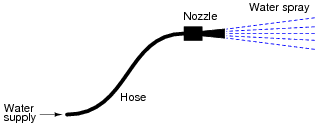• #### Question 5

Suppose you were to build this circuit and take measurements of current through the resistor and voltage across the resistor:Recording these numerical values in a table, the results look something like this:

 XXXXXXX XXXXXXX

 Current Voltage

 0.22 A 0.66 V

 0.47 A 1.42 V

 0.85 A 2.54 V

 1.05 A 3.16 V

 1.50 A 4.51 V

 1.80 A 5.41 V

 2.00 A 5.99 V

 2.51 A 7.49 V

Plot these figures on the following graph: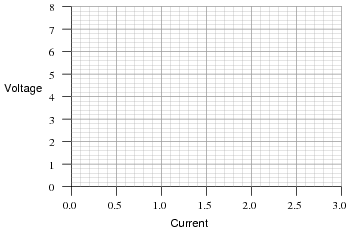What mathematical relationship do you see between voltage and current in this simple circuit?

• #### Question 6

Explain, step by step, how to calculate the amount of current (I) that will go through the resistor in this circuit: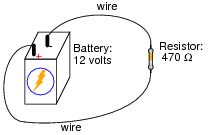• #### Question 7

Plot the relationships between voltage and current for resistors of three different values (1 Ω, 2 Ω, and 3 Ω), all on the same graph: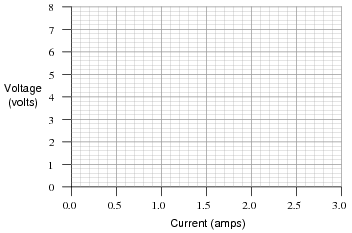What pattern do you see represented by your three plots? What relationship is there between the amount of resistance and the nature of the voltage/current function as it appears on the graph?

Advanced question: in calculus, the instantaneous rate-of-change of an (x,y) function is expressed through the use of the derivative notation: [dy/dx]. How would the derivative for each of these three plots be properly expressed using calculus notation? Explain how the derivatives of these functions relate to real electrical quantities.

• #### Question 8

What is the value of this resistor, in ohms (Ω)?• #### Question 9

A common saying about electricity is that it always takes the path of least resistance.” Explain how this proverb relates to the following circuit, where electric current from the battery encounters two alternate paths, one being less resistive than the other:• #### Question 10

One style of light bulb, very different from the “incandescent” design which works on the principle of a super-heated wire filament emitting light, is called a gas discharge tube. In this design of light bulb, light is produced by the direct “excitation” of gas molecules as electric current passes between two electrodes:Both types of light bulbs have interesting voltage/current plots, neither one being identical to the voltage/current plot of a resistor. First, the voltage/current plot for an incandescent light bulb: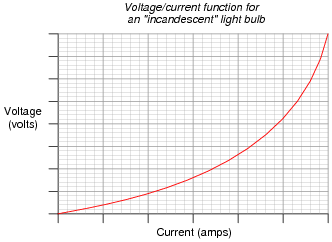Next, the voltage/current plot for a gas-discharge light bulb: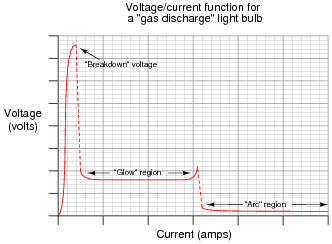Based on these two graphs, what can you say about the electrical resistance of each bulb type over its operating range?

• #### Question 11

Draw the schematic diagram for an experimental circuit to gather data necessary to plot the voltage/current graph of a gas discharge lamp.

• #### Question 12

What is negative resistance?

• #### Question 13

When an electric current passes through a conductor offering some electrical resistance, the temperature of that conductor increases above ambient. Why is this? Of what practical importance is this effect?

• #### Question 14

For a given amount of electric current, which resistor will dissipate the greatest amount of power: a small value (low-resistance) resistor, or a high value (high-resistance) resistor? Explain your answer.

• #### Question 15

Plot the relationship between power and current for a 2 Ω resistor on this graph: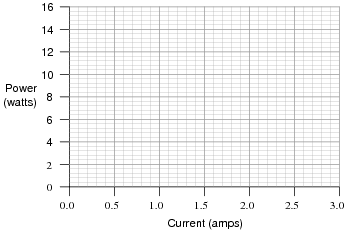What pattern do you see represented by plot? How does this compare with the graphical relationship between voltage and current for a resistor?

• #### Question 16

Shown here is a schematic diagram for a simple battery-powered flashlight:What could be modified about the circuit or its components to make the flashlight produce more light when turned on?

• #### Question 17

There are two basic Ohm’s Law equations: one relating voltage, current, and resistance; and the other relating voltage, current, and power (the latter equation is sometimes known as Joule’s Law rather than Ohm’s Law):

$$E=IR$$

$$P=IE$$

In electronics textbooks and reference books, you will find twelve different variations of these two equations, one solving for each variable in terms of a unique pair of two other variables. However, you need not memorize all twelve equations if you have the ability to algebraically manipulate the two simple equations shown above.

Demonstrate how algebra is used to derive the ten “other” forms of the two Ohm’s Law / Joule’s Law equations shown here.

• #### Question 18

In this circuit, three resistors receive the same amount of current (4 amps) from a single source. Calculate the amount of voltage “dropped” by each resistor, as well as the amount of power dissipated by each resistor:• #### Question 19

In this circuit, three resistors receive the same amount of voltage (24 volts) from a single source. Calculate the amount of current “drawn” by each resistor, as well as the amount of power dissipated by each resistor:• #### Question 20

The brightness of a light bulb - or the power dissipated by any electrical load, for that matter - may be varied by inserting a variable resistance in the circuit, like this:This method of electrical power control is not without its disadvantages, though. Consider an example where the circuit current is 5 amps, the variable resistance is 2 Ω, and the lamp drops 20 volts of voltage across its terminals. Calculate the power dissipated by the lamp, the power dissipated by the variable resistance, and the total power provided by the voltage source. Then, explain why this method of power control is not ideal.

• #### Question 21

A modern method of electrical power control involves inserting a fast-operating switch in-line with an electrical load, to switch power on and off to it very rapidly over time. Usually, a solid-state device such as a transistor is used: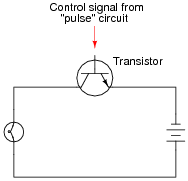This circuit has been greatly simplified from that of a real, pulse-control power circuit. Just the transistor is shown (and not the “pulse” circuit which is needed to command it to turn on and off) for simplicity. All you need to be aware of is the fact that the transistor operates like a simple, single-pole single-throw (SPST) switch, except that it is controlled by an electrical current rather than by a mechanical force, and that it is able to switch on and off millions of times per second without wear or fatigue.

If the transistor is pulsed on and off fast enough, power to the light bulb may be varied as smoothly as if controlled by a variable resistor. However, there is very little energy wasted when using a fast-switching transistor to control electrical power, unlike when a variable resistance is used for the same task. This mode of electrical power control is commonly referred to as Pulse-Width Modulation, or PWM.

Explain why PWM power control is much more efficient than controlling load power by using a series resistance.

• #### Question 22

What will happen to the brightness of the light bulb if the switch in this circuit is suddenly closed?• #### Question 23

What would happen if a wire having no resistance at all (0 Ω) were connected directly across the terminals of a 6-volt battery? How much current would result, according to Ohm’s Law?Suppose we were to short-circuit a 6-volt battery in the manner just described and measure 8 amps of current. Why don’t the calculated figures from the previous paragraph agree with the actual measurement?•mcg058 October 15, 2020